# HBSE 10th Class Maths Solutions Chapter 7 Coordinate Geometry Ex 7.2

Haryana State Board HBSE 10th Class Maths Solutions Chapter 7 Coordinate Geometry Ex 7.2 Textbook Exercise Questions and Answers.

## Haryana Board 10th Class Maths Solutions Chapter 7 Coordinate Geometry Ex 7.2

Question 1.
Find the co-ordinates of the point which divides the join of (- 1, 7) and (4, – 3) in the ratio 2 : 3.
Solution :
Let P(x, y) be the required point By section formula co-ordinates of P are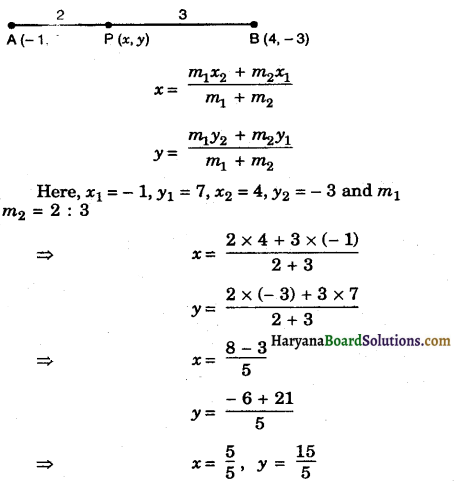⇒ x = 1, y = 3
Hence, the co-ordiantes of required point are (1, 3).Question 2.
Find the co-ordinates of the points
Solution :
Let P and Q be the points of trisection of AB.
i.e., AP = PQ = QB
Let AP = PQ = QB = K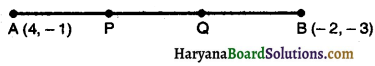PB = PQ + QB = 2K
AP + PQ = 2K
∴ AP : PB = K : 2K = 1 : 2
and, AQ : QB = 2K : K = 2 : 1.
Therefore, P divides AB in the ratio 1 : 2.
By section formula the co-ordinates of P are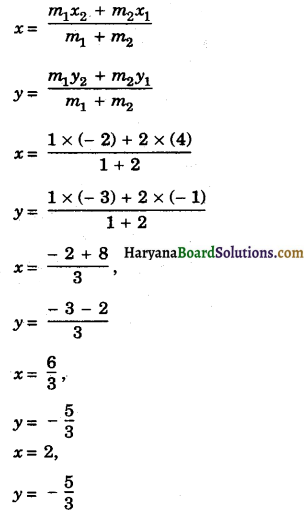So, the co-ordinates of p are (2, – $$\frac{5}{3}$$)
Now, Q divides AB in the ratio 2 : 1.
By section formula, co-ordinates of Q are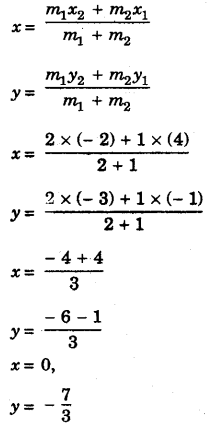So, the co-ordinates of Q are (0, – $$\frac{7}{3}$$)
Hence, co.-ordmates of trisection are (2, $$\frac{5}{3}$$) and (0, – $$\frac{7}{3}$$)).Question 3.
To conduct sports day tivities in your rectangular shaped school ground ABCD, lines have been drawn with chalk powder at a distance of 1 m each. 100 flower pots have been placed at a distance of 1 m from each other along AD, as shown in figure.

Niharika runs th the distance of AD on the 2nd line and posts a green flag. Preet runs th the distance AD on the eighth line and posts a red flag. What is the distance between both the flags ? If Rashmi has to post a blue flag exactly half way between the line segment joining the two flags, where should she post her flag?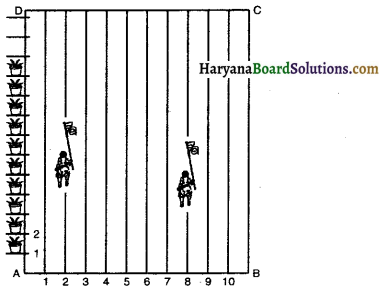Solution:
From the figure taking A as origin (0, 0), x-axis along AB and y-axis along AD, we will determine the co-ordinates of the green flag and red flag.
Now, the green flag in 2nd line and its distance parallel to AD.
AD = $$\frac{1}{4}$$ × 100 = 25 m.
So, the co-ordinates of green flag are (2, 25), we mark this position as G.
Similarly, co-ordinates of red flag = (8, 20), we mark this position as R.
Now,the distance between G and R = $$\sqrt{(8-2)^2+(20-25)^2}$$
= $$\sqrt{(6)^2+(-5)^2}$$
= $$\sqrt{36+25}$$
= $$\sqrt{61}]$$
Position of the blue flag is the mid point of GR.
Co-ordinates of blue flag = $$=\left(\frac{2+8}{2}, \frac{25+20}{2}\right)$$
= $$\left(\frac{10}{2}, \frac{45}{2}\right)$$
= (5, 22.5)
It means that blue flag is in the 5th line and at a distance of 22-5 m along the direction parallel to AD.
Hence, distance between G and R = $$\sqrt{61}]$$ m, and blue flag in 5th line at a distance of 22.5 m.Question 4.
Find the ratio in which the line segment joining the points (- 3, 10) and (6, – 8) is divided by (- 1, 6).
Solution :
Let the required ratio be m1 : m2
By section formula, we have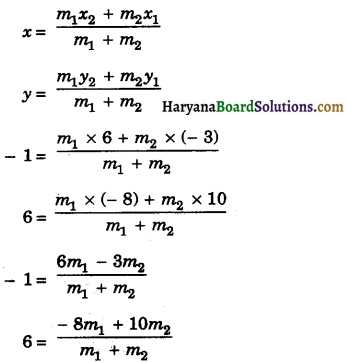Now, $$\frac{6 m_1-3 m_2}{m_1+m_2}$$ = – 1
⇒ 6m1 – 3m2 = – m1 – m2
⇒ 6m1 + m1 = 3 m2 – m2
⇒ 7m1 = 2m2
⇒ $$\frac{m_1}{m_2}=\frac{2}{7}$$
⇒ m1 : m2 = 2 : 7
Hence, the required ratio is 2 : 7.Question 5.
Find the ratio in which the line segment joining A(1, – 5) and B(- 4, 5) is divided by the x-axis. Also find the coordinates of the point of division.
Solution :
Let the required ratio be k : 1.
By section formula, we have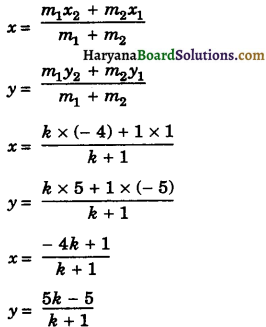Since, point lies on x-axis, so its ordinate is zero.
0 = $$\frac{5 k-5}{k+1}$$
5k – 5 = 0
k = $$\frac{5}{5}$$ = 1
So, the required ratio is 1 : 1.
Putting the value of k we get co-ordinates of the point of division.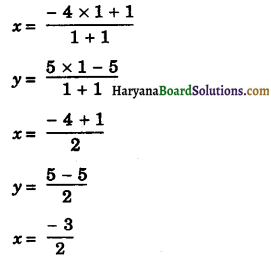y = 0
Hence, required ratio = 1: 1 and co-ordinates of the point of division = (- $$\frac{3}{2}$$, 0).Question 6.
If (1, 2), (4, y), (x, 6) and (3, 5) are the vertices of a parallelogram taken in order, find x and y.
Solution :
Let given vertices of a parallelogram be A(1, 2), B(4, y), C(x, 6) and D(3, 5). Join AC and BD.
We know that diagonals of a parallelogram bisect each other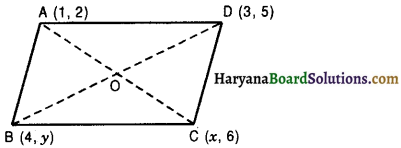So, O is the mid point of AC as well as that of BD.
Co-ordinates of mid point of AC = Co-ordinates of mid point of BD.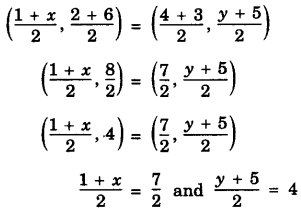1 + x = $$\frac{7 \times 2}{2}$$ and y + 5 = 8
x = 7 – 1 and y = 8 – 5
x = 6 and y = 3
Hence, x = 6 and y = 3

Question 7.
Find the co-ordinates of a point A, where AB is the diameter of a circle whose centre is (2, – 3) and B is (1, 4).
Solution :
Let the co-ordinates of point A be (x, y).
∵ O is the mid point of AB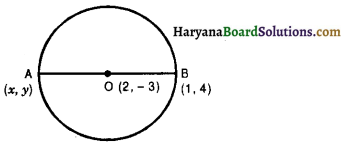Co-ordinates of O are 2 = $$\frac{x+1}{2}$$ and – 3 = $$\frac{y+4}{2}$$
⇒ 4 = x + 1 and – 6 = y + 4
⇒ 3 = x and – 10 = y
⇒ x = 3, and y = – 10
Hence, co-ordinates of point A are (3, – 10).Question 8.
If A and B are (- 2, – 2) and (2, – 4), respectively, find the co-ordinates of P such that AP = $$\frac{3}{7}$$ AB and P lies on the line segment AB.
Solution :
Let the co-ordinates of P be (x, y), we have
AP = $$\frac{3}{7}$$ AB
AP + PB = AB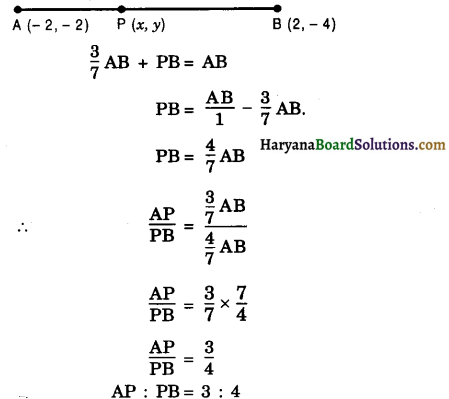By section formula, the co-ordinates of point P are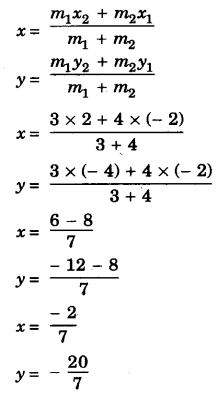Hence, co-ordinates of P are $$\left(-\frac{2}{7},-\frac{20}{7}\right)$$.Question 9.
Find the co-ordinates of the points which divide the line segment joining A(- 2, 2) and B(2, 8) into four equal parts.
Solution:
Let P, Q, R be three points that divides the line segment joining A(- 2, 2) and B(2, 8) in four equal parts.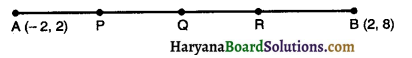Since Q is the mid point of AB.
Therefore, co-ordinates of point Q are $$\left(-\frac{2+2}{2}, \frac{2+8}{2}\right)$$ i.e. (0, 5)
Similarly, P is the mid point of AQ.
Therefore, co-ordinates of P are $$\left(\frac{-2+0}{2}, \frac{2+5}{2}\right)$$ i.e., (- 1, $$\frac{7}{2}$$)
and R is the mid point of QB.
Therefore, co-ordinates of R are $$\left(\frac{0+2}{2}, \frac{5+8}{2}\right)$$ i.e., (1, $$\frac{13}{2}$$)
Hence, the required co-ordinates are (- 1, $$\frac{7}{2}$$), (0, 5) and (1, $$\frac{13}{2}$$).

Question 10.
Find the area of a rhombus if its vertices are (3, 0), (4, 5), (- 1, 4) and (- 2, – 1) taken in order.
Solution :
Let coordinates of vertices of a rhombus be A(3, 0), B(4, 5), C(- 1, 4) and D(- 2, – 1), then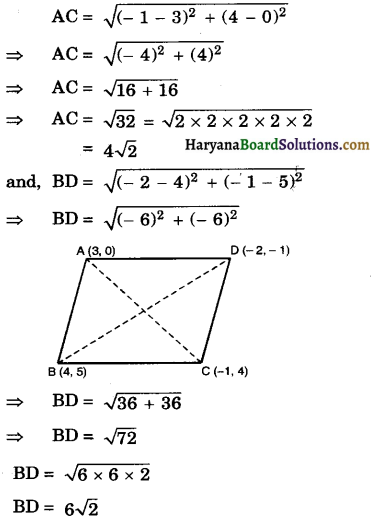Now, area of rhombus = $$\frac{1}{2}$$ (Product of diagonals)
= $$\frac{1}{2}$$ × AC × BD
= $$\frac{1}{2}$$ × 4√2 × 6√2
= 24 square units
Hence, area of rhombus = 24 square units.